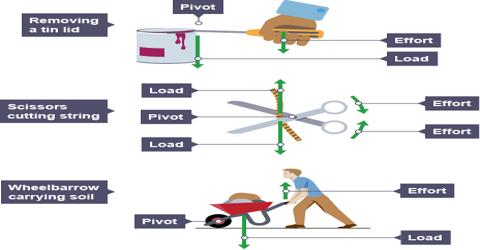# Lever Mechanism

Lever is a simple machine in which a strong bar can freely move upward and down and or rotate on a supporter. The lever is one of the most basic forms of a machine. It helps us lift loads with lesser effort.

The simple example of a lever is the act of lifting a heavy body by a crowbar which depends on a brick or stone to take support. Here, the heavy body is the weight and the force required to lift it is the force. The point at which the supporting body resists the strong bar to freely ascent and decent or to rotate is the pivot (fulcrum) of the lever. Lever helps raise or move a heavy body (load) with minimum application of force. Here the mechanical efficiency is-

Mechanical efficiency = weight/applied forceNow you are to understand how you can avail mechanical energy by using a lever. The principle of leaver is as follows:

Applied force x the length of force-arm = weight x the length of load-arm.

Here the length of force-arm is the distance between the point of applied force and the pivot. Similarly the distance from the pivot to the centre of load is the length of the load-arm.

Then the above principle is expressed by this equation:

load (weight)/applied force =  (the length of force/arm) x (the length of load/arm)